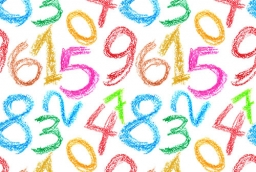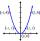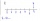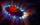# Two-digit number

Digit sum of thinking two-digit natural number is 11. When it exchanging a sequence of digits, given a number which is 27 less than the thinking number. Find out which number I think.

x =  74

### Step-by-step explanation:

x = 10•a+b
a+b = 11
10•b+a = x - 27

10a+b-x = 0
a+b = 11
a+10b-x = -27

a = 7
b = 4
x = 74

Our linear equations calculator calculates it.Did you find an error or inaccuracy? Feel free to write us. Thank you!Tips to related online calculators
Do you have a linear equation or system of equations and looking for its solution? Or do you have a quadratic equation?

## Related math problems and questions:

• Digit sumThe digit sum of the two-digit number is nine. When we turn figures and multiply by the original two-digit number, we get the number 2430. What is the original two-digit number?
• A number 4A number is made up of two digits. The sum of the digits is 11. if the digits are interchanged, the original number is increase by 9. Find the original number
• Unknown number 7Calculate unknown number whose 12th power when divided by the 9th power get a number 27 times greater than the unknown number. Determine the unknown number.
• The sumThe sum of the squares of two immediately following natural numbers is 1201. Find these numbers.
• I think numberI think number.When I add 841 to it and subtract 157, I get a number that is 22 greater than 996. What number I thinking?
• The sum 8The sum of two numbers is 21. If three times the smaller numbers is two less than twice the larger number, find the two numbers.
• Four-digit numbersFind four-digit numbers where all the digits are different. For numbers, the sum of the third and fourth digits is twice the sum of the first two digits, and the sum of the first and fourth digits is equal to the sum of the second and third digits. The di
• Digit sumFind the smallest natural number n, the digit sum of which is equal to 37.
• Unknown number 716% of the unknown number is by 21 less than unknown number itself. Determine the natural unknown number.
• Sum of three numbersThe sum of three numbers from which second number is 20% smaller than the first number and the third number is 25% smaller than the second number is 96. Determine this numbers.
• Two unknown numbersOne number is 2 less than a second number. Twice the second number is 14 less than 5 times the first. Find the two numbers.
• Digit sumDetermine for how many integers greater than 900 and less than 1,001 has digit sum digit of the digit sum number 1.
• Two-digit numberIn a two-digit number, the number of tens is three greater than the number of units. If we multiply the original number by a number written in the same digits but in reverse order, we get product 3 478. Find the original number.
• Seven timesWhich number seven times is just as higher as 27, how much is smaller than 29?
• Unknown number 4I think number. When I divided its square root by its 1/9, I get a number 1. What number am I thinking?
• NumbersDetermine the number of all positive integers less than 4183444 if each is divisible by 29, 7, 17. What is its sum?
• Two-digit numberI am a two-digit number less than 20. When I divided by three, then you get the rest 1 and when you divide me by four you get also rest 1. What number am I?